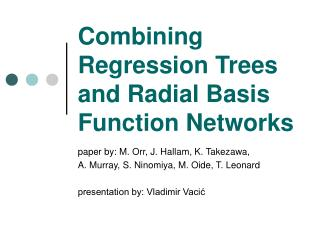DownloadDownload PresentationCombining Regression Trees and Radial Basis Function Networks

# Combining Regression Trees and Radial Basis Function Networks

Télécharger la présentation## Combining Regression Trees and Radial Basis Function Networks

- - - - - - - - - - - - - - - - - - - - - - - - - - - E N D - - - - - - - - - - - - - - - - - - - - - - - - - - -
##### Presentation Transcript

1. Combining Regression Trees and Radial Basis Function Networks paper by: M. Orr, J. Hallam, K. Takezawa, A. Murray, S. Ninomiya, M. Oide, T. Leonard presentation by: Vladimir Vacić

2. Contents: • Regression Trees • Radial Basis Function Neural Networks • Combining RTs and RBF NNs • Method • Experimental Results • Conclusion

3. Regression Trees Xik<b SL SR b

4. Radial Basis Function Neural Networks

5. Combining RTs and RBF NNs • RT generates candidate units for the RBF NN • RT specifies RBF centers and radii • RT influences the order in which candidate units are evaluated

6. Method Generating the regression tree: • recursively cut along the k dimensions • determine output for each node

7. Method Transforming tree nodes in RBFs: • center • radius

8. Method Selecting RBF units from the set of candidates: • necessary because so far we have not performed any pruning of the regression tree • too complex of a RBF runs into a risk of over-fitting • complex RBF is computationally expensive

9. Method Selecting RBF units from the set of candidates: • standard selection methods are forward selection, backward elimination, combination of the two, full combinatorial search… • problem with forward selection is that once choice may block subsequent informative choices

10. Method Using the trees to guide selection: • put the root node into the list of active nodes • for each node, consider the effect of adding one or both children and keeping or removing the parent • choose the combination which improves performance the most and update the active list • repeat

11. Method Calculating the weights: • least square minimization

12. Method Model selection criterion: • Bayesian information criterion (BIC) • BIC imposes a penalty for model complexity and hence leads to smaller networks

13. Method Note that so far we have had 2 free parameters : • p (controls the resulting network size) • a (controls the ratio of the RBF radii to corresponding hyper-rectangle size)

14. Experimental Results • the authors report that the best experimentally determined p and a on the training set do not always yield best performance on the test set • instead, they suggest using a set of best values for p and a from training and then find the best combination on the test set

15. Experimental Results • 2D sine wave problem • simulated circuit problem

16. Experimental Results Comparison with other learning methods: • linear least squares regression • k-nearest neighbor • ensembles of multilayer perceptrons • multilayer perceptrons trained using MCMC • multivariate adaptive regression splines (MARS) with bagging

17. Experimental Results Datasets: • DELVE dataset (non-linear, nigh noise, 8- and 32-dimensional examples), generated from simulated robotic arms • soybean classification into three classes (good, fair, poor) from digital images

18. Experimental Results DELVE, 8-dimensional examples:

19. Experimental Results DELVE, 32-dimensional examples:

20. Conclusion • improvement and analysis of previous work by Kubat • combining RTs and RBF NNs as a technique is competitive with some leading modern methods

21. Combining Regression Trees and Radial Basis Function Networks paper by: M. Orr, J. Hallam, K. Takezawa, A. Murray, S. Ninomiya, M. Oide, T. Leonard presentation by: Vladimir Vacić